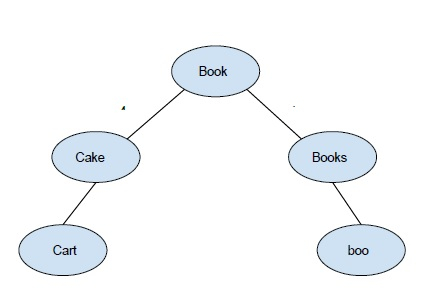# BK Tree Introduction in C++

BK tree or Burkhard tree is a form of a data structure usually used to perform spell checks based on Levenshtein distance. It is also used for string matching Autocorrect feature can be used making this data structure. Let's say we have some words in a dictionary and we need to check some other words for spelling errors. We need to have a collection of words that is close to the given word whose spelling is to be checked. For example, if we have the word “uck” The correct word can be (truck, duck, duck, suck). Therefore spelling mistakes can be corrected by deleting a word or adding a new word replacing a letter by an appropriate letter. Using the edit distance as a parameter and checking the spellings with the dictionary.

Like all other trees, the BK tree also consists of nodes and edges The nodes represent the words in a dictionary The edge contains some integer weights which gives us information about edit distance From one node to another.

Consider a dictionary with words { book, books, boo, cake, cape} −## BK Tree

Every note in BK Tree has exactly one child node with the same edit distance. If we encounter some collision in edit distance while inserting nodes, we will propagate the insertion process until we get the right child. Every insertion starts with the root node the root node can be any word. Till now we have learned what is Bk Tree. Now let's see how to find the correct closest word. First of all, we need to set tolerance value this tolerance value is nothing but maximum edit distance between my misspelled word and correct word.

To find the eligible correct word within the tolerance limit we use the process of iteration. But this has a higher complexity so now the BK tree comes into action as we know that each node in the binary tree is constructed based on its edit distance from the parent. So we can go directly from the root node to the specific node that lies within the tolerance limit. If TOL is the tolerance limit and edit distance of the current node from the misspelled node is dist. So now, we will iterate only those children that have edit distance in range.

[dist - TOL, dist+TOL], this reduces complexity to a larger extent.

## Example

Program to illustrate the working −

Live Demo

#include "bits/stdc++.h"
using namespace std;
#define MAXN 100
#define TOL 2
#define LEN 10
struct Node {
string word;
int next[2*LEN];
Node(string x):word(x){
for(int i=0; i<2*LEN; i++)
next[i] = 0;
}
Node() {}
};
Node RT;
Node tree[MAXN];
int ptr;
int min(int a, int b, int c) {
return min(a, min(b, c));
}
int editDistance(string& a,string& b) {
int m = a.length(), n = b.length();
int dp[m+1][n+1];
for (int i=0; i<=m; i++)
dp[i] = i;
for (int j=0; j<=n; j++)
dp[j] = j;
for (int i=1; i<=m; i++) {
for (int j=1; j<=n; j++) {
if (a[i-1] != b[j-1])
dp[i][j] = min( 1 + dp[i-1][j], 1 + dp[i][j-1], 1 + dp[i-1][j-1] );
else
dp[i][j] = dp[i-1][j-1];
}
}
return dp[m][n];
}
void insertValue(Node& root,Node& curr) {
if (root.word == "" ){
root = curr;
return;
}
int dist = editDistance(curr.word,root.word);
if (tree[root.next[dist]].word == ""){
ptr++;
tree[ptr] = curr;
root.next[dist] = ptr;
}
else{
insertValue(tree[root.next[dist]],curr);
}
}
vector <string> findCorrectSuggestions(Node& root,string& s){
vector <string> corrections;
if (root.word == "")
return corrections;
int dist = editDistance(root.word,s);
if (dist <= TOL) corrections.push_back(root.word);
int start = dist - TOL;
if (start < 0)
start = 1;
while (start < dist + TOL){
vector <string> temp = findCorrectSuggestions(tree[root.next[start]],s);
for (auto i : temp)
corrections.push_back(i);
start++;
}
return corrections;
}
int main(){
string dictionary[] = {"book","cake","cart","books", "boo" };
ptr = 0;
int size = sizeof(dictionary)/sizeof(string);
for(int i=0; i<size; i++){
Node tmp = Node(dictionary[i]);
insertValue(RT,tmp);
}
string word1 = "ok";
string word2 = "ke";
vector <string> match = findCorrectSuggestions(RT,word1);
cout<<"Correct words suggestions from dictionary for : "<<word1<<endl;
for (auto correctWords : match)
cout<<correctWords<<endl;
match = findCorrectSuggestions(RT,word2);
cout<<"Correct words suggestions from dictionary for : "<<word2<<endl;
for (auto correctWords : match)
cout<<correctWords<<endl;
return 0;
}

## Output

Correct words suggestions from dictionary for : ok
book
boo
Correct words suggestions from dictionary for : ke
cake

Updated on: 05-Aug-2020

171 Views